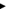Using Flash'sMathobject, you can round to the nearest whole number using theMath.roundcommand. You may, however, prefer to round to different decimal places. This TechNote offers a technique for rounding to a specified decimal place.

Rounding to the hundredth decimal

Rounding to the nearest decimal simply involves inserting code in the Actions panel, as follows:

 1 Open your movie in Flash. 2 Select a Frame or an Instance. 3 Choose Windows > Panels > Actions. 4 Add this ActionScript in your Actions Panel to round to the hundredth decimal place, replacing yourNumber with the value you would like to round: value= int((yourNumber)*100)/100;

How the code works

The number used in the example below is 20 divided by 7, which equates to 2.857142857142857.

value= int((20/7)*100)/100;int(20/7)returns the value of 2 by removing the decimal places off the number created by the calculation in parentheses so that it appears as a whole number.Multiplying that result by 100 gives 285. Note: This is due to the fact that theintfunction does not erase the decimals; it just does not show them.Dividing 285 by 100 gives 2.85. You have now rounded to the nearest hundredth.

Rounding to other decimal places

If you prefer to round to a decimal other than to the hundredth, you can easily modify the code. Below are examples of code that will round (20/7) to different decimals:

 Code Number of Decimal Places Example Results value= Math.round(20/7); none 3 value= int((20/7)*10)/10; 1 2.8 value= int((20/7)*100)/100; 2 2.85 value= int((20/7)*1000)/1000; 3 2.857 value= int((20/7)*10000)/10000; 4 2.85 71Twitter™ and Facebook posts are not covered under the terms of Creative Commons.